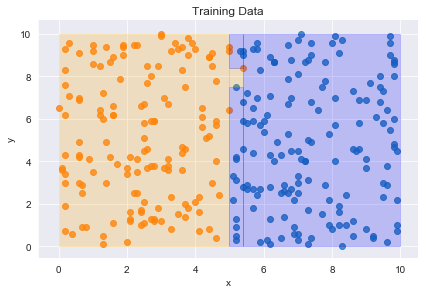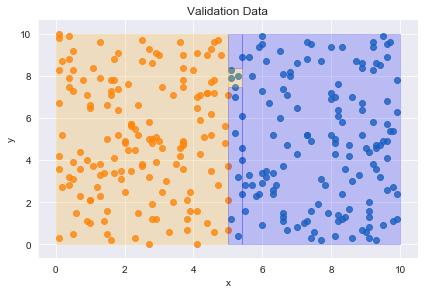In :
import numpy as np
import pandas as pd

from pprint import pprint

from decision_tree_functions import decision_tree_algorithm, make_predictions, calculate_accuracy
from helper_functions import generate_data, create_plot, train_test_split


# 1. Post-Pruning¶

In :
np.random.seed(0)
df_train = generate_data(n=300, specific_outliers=[(5.4, 8.4)])
create_plot(df_train, tree, title="Training Data")

np.random.seed(7)
df_val = generate_data(n=300)
create_plot(df_val, tree, title="Validation Data")In :
tree = {'x <= 5.0': [True, False]}

In :
def post_pruning(tree, df_train, df_val):

question = list(tree.keys())

# base case
leaf = df_train.label.value_counts().index
errors_leaf = sum(df_val.label != leaf)
errors_decision_node = sum(df_val.label != make_predictions(df_val, tree))

if errors_leaf <= errors_decision_node:
return leaf
else:
return tree

# recursive part
else:

return tree

In [ ]: